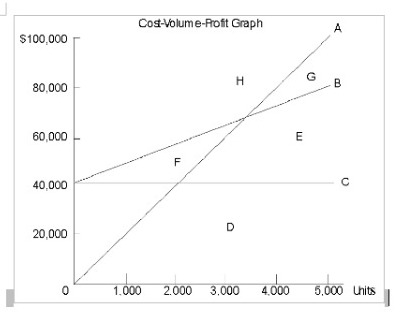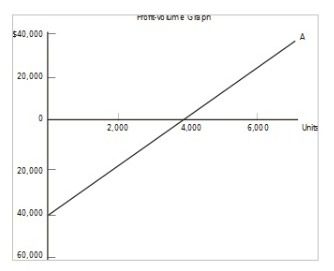# Accounting Practical Solved Assignment Solution Sample

QUESTION

Part I: Multiple Choice- 3 Points each (60 points)

Please place your answer legibly in capital letters at the space provided to the left of each question.

__________1. The break-even point is that level of activity where:
A. total revenue equals total cost.
B. variable cost equals fixed cost.
C. total contribution margin equals the sum of variable cost plus fixed cost.
D. sales revenue equals total variable cost.

__________2. The unit contribution margin is calculated as the difference between:
A. selling price and fixed cost per unit.
B. selling price and variable cost per unit.
C. selling price and product cost per unit.
D. fixed cost per unit and variable cost per unit.

__________3. Which of the following would take place if a company experienced an increase in fixed costs?
A. Net income would increase.
B. The break-even point would increase.
C. The contribution margin would increase.
D. The contribution margin would decrease.

__________4. At a volume of 20,000 units, Dries reported sales revenues of \$1,000,000, variable costs of \$300,000, and fixed costs of \$260,000. The company’s contribution margin per unit is:
A. \$22.
B. \$28.
C. \$35.
D. \$37.

__________5. At a volume of 20,000 units, Dries reported sales revenues of \$1,000,000, variable costs of \$300,000, and fixed costs of \$260,000. The company’s break-even point in units is:
A. 7,027 (rounded).
B. 8,667 (rounded).
C. 9,286 (rounded).
D. 7,429 (rounded).

__________6. Grey, Inc. sells a single product for \$20. Variable costs are \$8 per unit and fixed costs total \$120,000 at a volume level of 5,000 units. Assuming that fixed costs do not change, Green’s break-even sales would be:
A. \$160,000.
B. \$200,000.
C. \$300,000.
D. \$480,000.

__________7. The contribution-margin ratio is:
A. the difference between the selling price and the variable cost per unit.
B. fixed cost per unit divided by variable cost per unit.
C. variable cost per unit divided by the selling price.
D. unit contribution margin divided by the selling price.

__________8. The following information relates to Day Company:

 Sales revenue \$12,000,000 Contribution margin 4,800,000 Net income 800,000

Day’s operating leverage factor is:

A. 6.000.

B. 0.167.

C. 0.400.

D. 2.500.__________9. Refer to the figure above. Line A is the:
A. total revenue line.
B. fixed cost line.
C. variable cost line.
D. total cost line.

__________10. Lamar & Co., makes and sells two types of shoes, Plain and Fancy. Data concerning these products are as follows:

 Plain Fancy Unit selling price \$20.00 \$35.00 Variable cost per unit 12.00 24.50

Sixty percent of the unit sales are Plain, and annual fixed expenses are \$45,000.

Assuming that the sales mix remains constant, the number of units of Plain that the company must sell to break even is:

A. 2,000.

B. 3,000.

C. 3,375.

D. 5,000.

Use the following to answer questions 11-12:__________11. Line A is the:

A. fixed cost line.

B. variable cost line.

C. profit line.

D. total revenue line.

__________12. The triangular area between the horizontal axis and Line A, to the right of 4,000, represents:

A. fixed cost.

B. variable cost.

C. profit.

D. loss.

__________13. A recent income statement of Oslo Corporation reported the following data:

 Units sold 8,000 Sales revenue \$7,200,000 Variable costs 4,000,000 Fixed costs 1,600,000

If the company desired to earn a target net profit of \$480,000, it would have to sell:

A. 1,200 units.

B. 2,800 units.

C. 4,000 units.

D. 5,200 units.

__________14. A budget serves as a benchmark against which:

A. actual results can be compared.

B. allocated results can be compared.

C. actual results become inconsequential.

D. allocated results become inconsequential.

__________15. A manufacturing firm would begin preparation of its master budget by constructing a:

A. sales budget.

B. production budget.

C. cash budget.

D. capital budget.

______16. The budgeted income statement, budgeted balance sheet, and budgeted statement of cash flows comprise:
A. the final portion of the master budget.
B. the depiction of an organization’s overall actual financial results.
C. the first step of the master budget.
D. the portion of the master budget prepared after the sales forecast and before the remainder of the operational budgets.

______17. Bird plans to sell 5,000 units each quarter next year. During the first two quarters each unit will sell for \$12; during the last two quarters the sales price will increase \$1.50 per unit. What is Bird’s estimated sales revenue for next year?
A. \$240,000.
B. \$255,000.
C. \$270,000.
D. \$244,000.

______18. Quattro began operations in April of this year. It makes all sales on account, subject to the following collection pattern: 30% are collected in the month of sale; 60% are collected in the first month after sale; and 10% are collected in the second month after sale. If sales for April, May, and June were \$60,000, \$80,000, and \$70,000, respectively, what were the firm’s budgeted collections for April?
A. \$18,000.
B. \$21,000.
C. \$60,000.
D. \$65,000.

______19. Digregory makes all purchases on account, subject to the following payment pattern:
Paid in the month of purchase: 30%
Paid in the first month following purchase: 60%
Paid in the second month following purchase: 10%
If purchases for January, February, and March were \$200,000, \$180,000, and \$230,000, respectively, what were the firm’s budgeted payments in March?
A. \$69,000.
B. \$138,000.
C. \$177,000.
D. \$197,000.

______20. Coleman, Inc., anticipates sales of 50,000 units, 48,000 units, and 51,000 units in July, August, and September, respectively. Company policy is to maintain an ending finished-goods inventory equal to 40% of the following month’s sales. On the basis of this information, how many units would the company plan to produce in August?

A. 46,800.

B. 49,200.

C. 49,800.

D. 52,200.

Part II: Problem I (40 points)

Quicksand Company has set the following standards for one unit of product:

Direct material

Quantity: 6.2 pounds per unit

Price per pound: \$11 per pound

Direct labor

Quantity: 6 hours per unit

Rate per hour: \$23 per hour

Actual costs incurred in the production of 2,800 units were as follows:

Direct material: \$194,350 (\$11.50 per pound)

Direct labor: \$393,750 (\$22.50 per hour)

All materials purchased were consumed during the period.

Required:

1. Calculate the direct-material price variance.

2. Calculate the direct-material quantity variance.

3. Calculate the direct-labor rate variance.

4. Calculate the direct-labor rate efficiency variance.

Part 1

1.) A

2.) B

3. B

4.) C

5.) D

6.) B

7.) D

8.) A

9.) A

10.) C

11.) C

12.) C

13.) D

14.) A

15.) A

16.) A

17.) B

18.) A

19.) D

20.) B

Part 2

1.

Direct Material Price Variance= (Standard Price per unit- Actual Price per unit)x Actual Quantity purchased

= (\$11-\$11.50)x\$194350/11.50

= \$ 8450 Unfavorable

2.

Direct Material Quantity Variance= (Standard quantity – Actual quantity consumed unit) x Standard Price per unit

= (6.2x 2800)-((\$194350/11.50)) x \$11

= \$ 5060 Favorable

3.

Direct Labor rate variance= (Standard labor rate per hour- actual labor rate per hour x Actual labor hours consumed

= (\$23- \$22.50) x ( \$393750/22.50)

= \$ 8750 Favorable

4.

Direct Labor rate efficiency variance= (Standard labor hours- actual labor hours) x Standard Labor rate

= ((6×2800)-(\$ 393750/22.50) x\$23

= \$16100 Unfavorable

Looking for best Accounting Assignment Help. Whatsapp us at +16469488918 or chat with our chat representative showing on lower right corner or order from here. You can also take help from our Live Assignment helper for any exam or live assignment related assistance.